# Performance of Sparse Recovery Using L1 Minimization¶

Important: Please read the installation page for details about how to install the toolboxes. $\newcommand{\dotp}{\langle #1, #2 \rangle}$ $\newcommand{\enscond}{\lbrace #1, #2 \rbrace}$ $\newcommand{\pd}{ \frac{ \partial #1}{\partial #2} }$ $\newcommand{\umin}{\underset{#1}{\min}\;}$ $\newcommand{\umax}{\underset{#1}{\max}\;}$ $\newcommand{\umin}{\underset{#1}{\min}\;}$ $\newcommand{\uargmin}{\underset{#1}{argmin}\;}$ $\newcommand{\norm}{\|#1\|}$ $\newcommand{\abs}{\left|#1\right|}$ $\newcommand{\choice}{ \left\{ \begin{array}{l} #1 \end{array} \right. }$ $\newcommand{\pa}{\left(#1\right)}$ $\newcommand{\diag}{{diag}\left( #1 \right)}$ $\newcommand{\qandq}{\quad\text{and}\quad}$ $\newcommand{\qwhereq}{\quad\text{where}\quad}$ $\newcommand{\qifq}{ \quad \text{if} \quad }$ $\newcommand{\qarrq}{ \quad \Longrightarrow \quad }$ $\newcommand{\ZZ}{\mathbb{Z}}$ $\newcommand{\CC}{\mathbb{C}}$ $\newcommand{\RR}{\mathbb{R}}$ $\newcommand{\EE}{\mathbb{E}}$ $\newcommand{\Zz}{\mathcal{Z}}$ $\newcommand{\Ww}{\mathcal{W}}$ $\newcommand{\Vv}{\mathcal{V}}$ $\newcommand{\Nn}{\mathcal{N}}$ $\newcommand{\NN}{\mathcal{N}}$ $\newcommand{\Hh}{\mathcal{H}}$ $\newcommand{\Bb}{\mathcal{B}}$ $\newcommand{\Ee}{\mathcal{E}}$ $\newcommand{\Cc}{\mathcal{C}}$ $\newcommand{\Gg}{\mathcal{G}}$ $\newcommand{\Ss}{\mathcal{S}}$ $\newcommand{\Pp}{\mathcal{P}}$ $\newcommand{\Ff}{\mathcal{F}}$ $\newcommand{\Xx}{\mathcal{X}}$ $\newcommand{\Mm}{\mathcal{M}}$ $\newcommand{\Ii}{\mathcal{I}}$ $\newcommand{\Dd}{\mathcal{D}}$ $\newcommand{\Ll}{\mathcal{L}}$ $\newcommand{\Tt}{\mathcal{T}}$ $\newcommand{\si}{\sigma}$ $\newcommand{\al}{\alpha}$ $\newcommand{\la}{\lambda}$ $\newcommand{\ga}{\gamma}$ $\newcommand{\Ga}{\Gamma}$ $\newcommand{\La}{\Lambda}$ $\newcommand{\si}{\sigma}$ $\newcommand{\Si}{\Sigma}$ $\newcommand{\be}{\beta}$ $\newcommand{\de}{\delta}$ $\newcommand{\De}{\Delta}$ $\newcommand{\phi}{\varphi}$ $\newcommand{\th}{\theta}$ $\newcommand{\om}{\omega}$ $\newcommand{\Om}{\Omega}$

This tour explores theoritical garantees for the performance of recovery using $\ell^1$ minimization.

In :
from __future__ import division

import numpy as np
import scipy as scp
import pylab as pyl
import matplotlib.pyplot as plt

from nt_toolbox.general import *
from nt_toolbox.signal import *

import warnings
warnings.filterwarnings('ignore')

%matplotlib inline


## Sparse $\ell^1$ Recovery¶

We consider the inverse problem of estimating an unknown signal $x_0 \in \RR^N$ from noisy measurements $y=\Phi x_0 + w \in \RR^P$ where $\Phi \in \RR^{P \times N}$ is a measurement matrix with $P \leq N$, and $w$ is some noise.

This tour is focused on recovery using $\ell^1$ minimization $$x^\star \in \uargmin{x \in \RR^N} \frac{1}{2}\norm{y-\Phi x}^2 + \la \norm{x}_1.$$

Where there is no noise, we consider the problem $\Pp(y)$ $$x^\star \in \uargmin{\Phi x = y} \norm{x}_1.$$

We are not concerned here about the actual way to solve this convex problem (see the other numerical tours on sparse regularization) but rather on the theoritical analysis of wether $x^\star$ is close to $x_0$.

More precisely, we consider the following three key properties

• Noiseless identifiability: $x_0$ is the unique solution of $\Pp(y)$ for $y=\Phi x_0$.
• Robustess to small noise: one has $\norm{x^\star - x_0} = O(\norm{w})$ for $y=\Phi x_0+w$ if $\norm{w}$ is smaller than an arbitrary small constant that depends on $x_0$ if $\la$ is well chosen according to $\norm{w}$.
• Robustess to bounded noise: same as above, but $\norm{w}$ can be arbitrary.

Note that noise robustness implies identifiability, but the converse is not true in general.

## Coherence Criteria¶

The simplest criteria for identifiality are based on the coherence of the matrix $\Phi$ and depends only on the sparsity $\norm{x_0}_0$ of the original signal. This criteria is thus not very precise and gives very pessimistic bounds.

The coherence of the matrix $\Phi = ( \phi_i )_{i=1}^N \in \RR^{P \times N}$ with unit norm colum $\norm{\phi_i}=1$ is $$\mu(\Phi) = \umax{i \neq j} \abs{\dotp{\phi_i}{\phi_j}}.$$

Compute the correlation matrix (remove the diagonal of 1's).

In :
remove_diag = lambda C: C-np.diag(np.diag(C))
Correlation = lambda Phi: remove_diag(abs(np.dot(np.transpose(Phi),Phi)))


Compute the coherence $\mu(\Phi)$.

In :
mu = lambda Phi: np.max(Correlation(Phi))


The condition $$\norm{x_0}_0 < \frac{1}{2}\pa{1 + \frac{1}{\mu(\Phi)}}$$ implies that $x_0$ is identifiable, and also implies to robustess to small and bounded noise.

Equivalently, this condition can be written as $\text{Coh}(\norm{x_0}_0)<1$ where $$\text{Coh}(k) = \frac{k \mu(\Phi)}{ 1 - (k-1)\mu(\Phi) }$$

In :
Coh = lambda Phi, k: (k*mu(Phi))/(1 - (k-1)*mu(Phi))


Generate a matrix with random unit columns in $\RR^P$.

In :
from numpy import random

normalize = lambda Phi:  Phi/np.tile(np.sqrt(np.sum(Phi**2,0)), (np.shape(Phi),1))
PhiRand = lambda P, N: normalize(random.randn(P, N))
Phi = PhiRand(250, 1000)


Compute the coherence and the maximum possible sparsity to ensure recovery using the coherence bound.

In :
c = mu(Phi)
print("Coherence:    %.2f" %c)
print("Sparsity max: %d" %np.floor(1/2*(1 + 1/c)))

Coherence:    0.30
Sparsity max: 2


Exercise 1

Display how the average coherence of a random matrix decays with the redundancy $\eta = P/N$ of the matrix $\Phi$. Can you derive an empirical law between $P$ and the maximal sparsity?

In :
run -i nt_solutions/sparsity_6_l1_recovery/exo1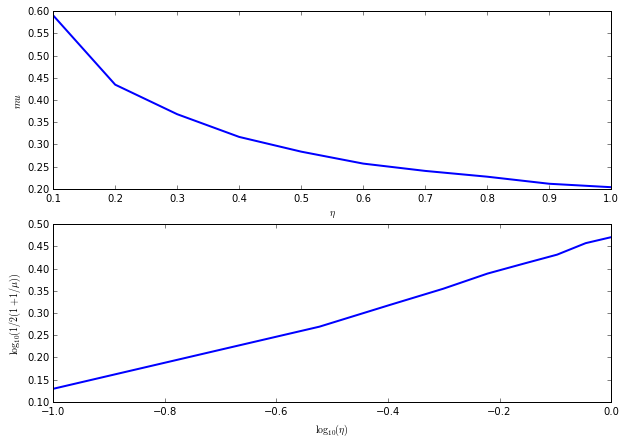In :
## Insert your code here.


## Support and Sign-based Criteria¶

In the following we will consider the support $$\text{supp}(x_0) = \enscond{i}{x_0(i) \neq 0}$$ of the vector $x_0$. The co-support is its complementary $I^c$.

In :
supp   = lambda s: np.where(abs(s) > 1e-5)
cosupp = lambda s: np.where(abs(s) < 1e-5)


Given some support $I \subset \{0,\ldots,N-1\}$, we will denote as $\Phi = (\phi_m)_{m \in I} \in \RR^{N \times \abs{I}}$ the sub-matrix extracted from $\Phi$ using the columns indexed by $I$.

J.J. Fuchs introduces a criteria $F$ for identifiability that depends on the sign of $x_0$.

J.J. Fuchs. Recovery of exact sparse representations in the presence of bounded noise. IEEE Trans. Inform. Theory, 51(10), p. 3601-3608, 2005

Under the condition that $\Phi_I$ has full rank, the $F$ measure of a sign vector $s \in \{+1,0,-1\}^N$ with $\text{supp}(s)=I$ reads $$\text{F}(s) = \norm{ \Psi_I s_I }_\infty \qwhereq \Psi_I = \Phi_{I^c}^* \Phi_I^{+,*}$$ where $A^+ = (A^* A)^{-1} A^*$ is the pseudo inverse of a matrix $A$.

The condition $$\text{F}(\text{sign}(x_0))<1$$ implies that $x_0$ is identifiable, and also implies to robustess to small noise. It does not however imply robustess to a bounded noise.

Compute $\Psi_I$ matrix.

In :
from numpy import linalg

PsiI = lambda Phi,I: np.dot(np.transpose(Phi[:,np.setdiff1d(np.arange(0,np.shape(Phi)), I)]),np.transpose(linalg.pinv(Phi[:,I])))


Compute $\text{F}(s)$.

In :
F = lambda Phi,s: linalg.norm(np.dot(PsiI(Phi, supp(s)),s[supp(s)]), np.float("inf"))


The Exact Recovery Criterion (ERC) of a support $I$, introduced by Tropp in

J. A. Tropp. Just relax: Convex programming methods for identifying sparse signals. IEEE Trans. Inform. Theory, vol. 52, num. 3, pp. 1030-1051, Mar. 2006.

Under the condition that $\Phi_I$ has full rank, this condition reads $$\text{ERC}(I) = \norm{\Psi_{I}}_{\infty,\infty} = \umax{j \in I^c} \norm{ \Phi_I^+ \phi_j }_1.$$ where $\norm{A}_{\infty,\infty}$ is the $\ell^\infty-\ell^\infty$ operator norm of a matrix $A$.

In :
erc = lambda Phi, I: linalg.norm(PsiI(Phi, I), np.float("inf"))


The condition $$\text{ERC}(\text{supp}(x_0))<1$$ implies that $x_0$ is identifiable, and also implies to robustess to small and bounded noise.

One can prove that the ERC is the maximum of the F criterion for all signs of the given support $$\text{ERC}(I) = \umax{ s, \text{supp}(s) \subset I } \text{F}(s).$$

The weak-ERC is an approximation of the ERC using only the correlation matrix $$\text{w-ERC}(I) = \frac{ \umax{j \in I^c} \sum_{i \in I} \abs{\dotp{\phi_i}{\phi_j}} }{ 1-\umax{j \in I} \sum_{i \neq j \in I} \abs{\dotp{\phi_i}{\phi_j}} }$$

In :
g = lambda C,I: np.sum(C[:,I], 1)
werc_g = lambda g,I,J: np.max(g[J])/(1-np.max(g[I]))
werc = lambda Phi,I: werc_g(g(Correlation(Phi), I), I, np.setdiff1d(np.arange(0,np.shape(Phi)), I))


One has, if $\text{w-ERC}(I)>0$, for $I = \text{supp}(s)$, $$\text{F}(s) \leq \text{ERC}(I) \leq \text{w-ERC}(I) \leq \text{Coh}(\abs{I}).$$

This shows in particular that the condition $$\text{w-ERC}(\text{supp}(x_0))<1$$ implies identifiability and robustess to small and bounded noise.

Exercise 2

Show that this inequality holds on a given matrix. What can you conclude about the sharpness of these criteria ?

In :
run -i nt_solutions/sparsity_6_l1_recovery/exo2

N = 2000, P = 1990, |I| = 6
F(s)     = 0.21
ERC(I)   = 0.23
w-ERC(s) = 0.26
Coh(|s|) = 1.47

In :
## Insert your code here.

N = 2000
P = N-10
Phi = PhiRand(N, P)
s = np.zeros(N)
s[:6] = 1
I = supp(s)
k = len(I)

print("N = %d, P = %d, |I| = %d" %(N,P,k))
print("F(s)     = %.2f"  %F(Phi, s))
print("ERC(I)   = %.2f"  %erc(Phi, I))
print("w-ERC(s) = %.2f" %werc(Phi, I))
print("Coh(|s|) = %.2f" %Coh(Phi, k))

N = 2000, P = 1990, |I| = 6
F(s)     = 0.20
ERC(I)   = 0.24
w-ERC(s) = 0.26
Coh(|s|) = 1.44


Exercise 3

For a given matrix $\Phi$ generated using PhiRand, draw as a function of the sparsity $k$ the probability that a random sign vector $s$ of sparsity $\norm{s}_0=k$ satisfies the conditions $\text{F}(x_0)<1$, $\text{ERC}(x_0)<1$ and $\text{w-ERC}(x_0)<1$

In :
run -i nt_solutions/sparsity_6_l1_recovery/exo3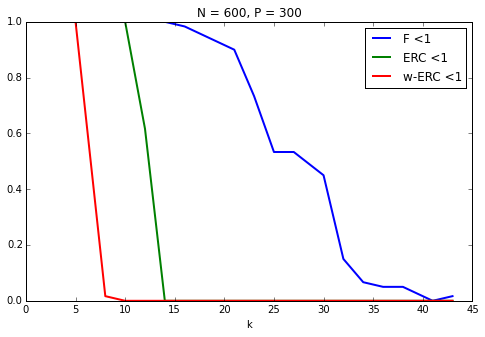In :
## Insert your code here.

N = 600
P = N/2
Phi = PhiRand(P, N)
klist = np.round(np.linspace(1, P/7., 20))
ntrials = 60
proba = np.zeros([len(klist),3])

for i in range(len(klist)):
proba[i,:3] = 0
k = klist[i]
for j in range(ntrials):
s = np.zeros(N)
I = random.permutation(N)
I = I[:k]
s[I] = np.sign(random.randn(k, 1))
proba[i, 0] = proba[i, 0] + (F(Phi, s) < 1)
proba[i, 1] = proba[i, 1] + (erc(Phi, I) < 1)
proba[i, 2] = proba[i, 2] + (werc(Phi, I) > 0)*(werc(Phi, I) < 1)

plt.figure(figsize = (8,5))
plt.plot(klist, proba/ntrials, linewidth=2)
plt.xlabel("k")
plt.legend(['F <1', 'ERC <1', 'w-ERC <1'])
plt.title("N = %d, P = %d" %(N,P))
plt.show()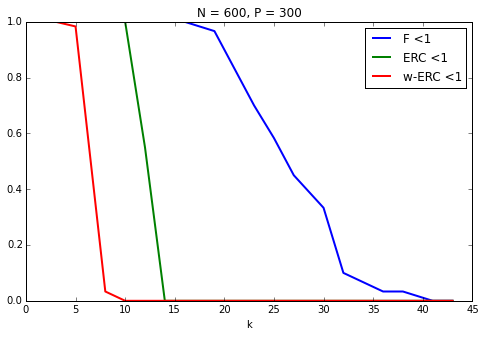## Restricted Isometry Criteria¶

The restricted isometry constants $\de_k^1,\de_k^2$ of a matrix $\Phi$ are the smallest $\de^1,\de^2$ that satisfy $$\forall x \in \RR^N, \quad \norm{x}_0 \leq k \qarrq (1-\de^1)\norm{x}^2 \leq \norm{\Phi x}^2 \leq (1+\de^2)\norm{x}^2.$$

E. Candes shows in

E. J. Cand s. The restricted isometry property and its implications for compressed sensing. Compte Rendus de l'Academie des Sciences, Paris, Serie I, 346 589-592

that if $$\de_{2k} \leq \sqrt{2}-1 ,$$ then $\norm{x_0} \leq k$ implies identifiability as well as robustness to small and bounded noise.

The stability constant $\la^1(A), \la^2(A)$ of a matrix $A = \Phi_I$ extracted from $\Phi$ is the smallest $\tilde \la_1,\tilde \la_2$ such that $$\forall \al \in \RR^{\abs{I}}, \quad (1-\tilde\la_1)\norm{\al}^2 \leq \norm{A \al}^2 \leq (1+\tilde \la_2)\norm{\al}^2.$$

These constants $\la^1(A), \la^2(A)$ are easily computed from the largest and smallest eigenvalues of $A^* A \in \RR^{\abs{I} \times \abs{I}}$

In :
minmax = lambda v: (1-np.min(v), max(v)-1)
ric = lambda A: minmax(linalg.eig(np.dot(np.transpose(A),A)))


The restricted isometry constant of $\Phi$ are computed as the largest stability constants of extracted matrices $$\de^\ell_k = \umax{ \abs{I}=k } \la^\ell( \Phi_I ).$$

The eigenvalues of $\Phi$ are essentially contained in the interval $[a,b]$ where $a=(1-\sqrt{\be})^2$ and $b=(1+\sqrt{\be})^2$ with $\beta = k/P$ More precisely, as $k=\be P$ tends to infinity, the distribution of the eigenvalues tends to the Marcenko-Pastur law $f_\be(\la) = \frac{1}{2\pi \be \la}\sqrt{ (\la-b)^+ (a-\la)^+ }.$

Exercise 4

Display, for an increasing value of $k$ the histogram of repartition of the eigenvalues $A^* A$ where $A$ is a Gaussian matrix of size $(P,k)$ and variance $1/P$. For this, accumulate the eigenvalues for many realizations of $A$.

In :
run -i nt_solutions/sparsity_6_l1_recovery/exo4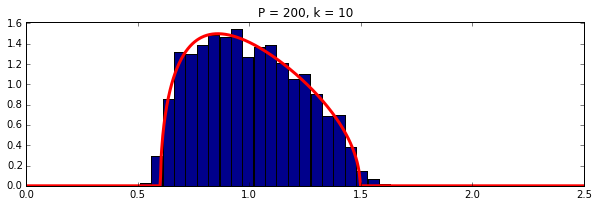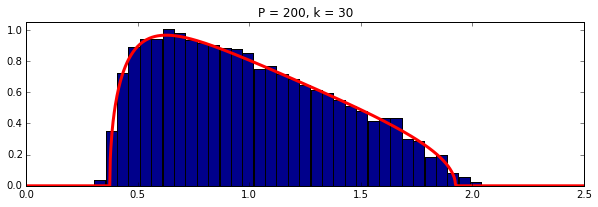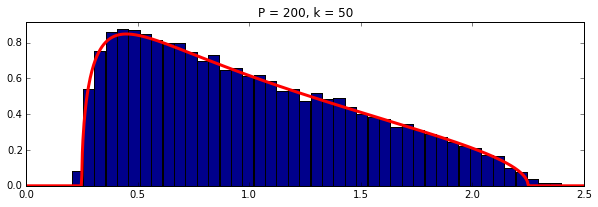In :
## Insert your code here.


Exercise 5

Estimate numerically lower bound on $\de_k^1,\de_k^2$ by Monte-Carlo sampling of sub-matrices.

In :
run -i nt_solutions/sparsity_6_l1_recovery/exo5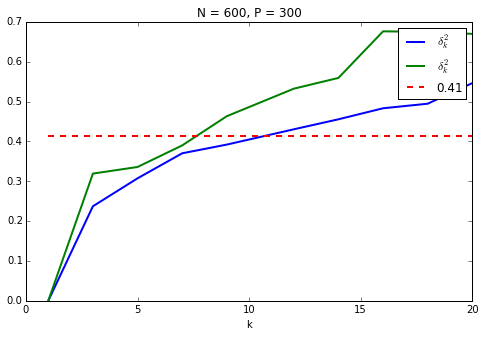In :
## Insert your code here.


## Sparse Spikes Deconvolution¶

We now consider a convolution dictionary $\Phi$. Such a dictionary is used with sparse regulariz

Second derivative of Gaussian kernel $g$ with a given variance $\si^2$.

In :
sigma = 6
g = lambda x: (1-x**2/sigma**2)*np.exp(-x**2/(2*sigma**2))


Create a matrix $\Phi$ so that $\Phi x = g \star x$ with periodic boundary conditions.

In :
P = 1024
[Y, X] = np.meshgrid(np.arange(0,P), np.arange(0,P))
Phi = normalize(g((X - Y + P/2.)%P-P/2.))


To improve the conditionning of the dictionary, we sub-sample its atoms, so that $P = \eta N > N$.

In :
eta = 2
N = P/eta
Phi = Phi[:,::eta]


Plot the correlation function associated to the filter. Can you determine the value of the coherence $\mu(\Phi)$?

In :
c = np.dot(np.transpose(Phi),Phi)
c = np.abs(c[:,np.shape(c)/2])

plt.figure(figsize=(8,5))
plt.plot(c[len(c)/2 - 50:len(c)/2 + 50], '.-')
plt.show()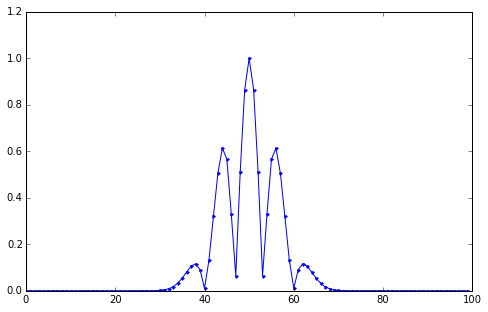Create a data a sparse $x_0$ with two diracs of opposite signes with spacing $d$.

In :
twosparse = lambda d: np.roll(np.concatenate(([1.], np.zeros(d), [-1.], np.zeros(N-d-2))), int(N/2 - d/2))


Display $x_0$ and $\Phi x_0$.

In :
x0 = twosparse(50)

plt.figure(figsize=(10,7))
plt.subplot(2, 1, 1)
plt.plot(x0, 'r', linewidth=2)
plt.subplot(2, 1, 2)
plt.plot(Phi*x0, 'b', linewidth=2)
plt.show()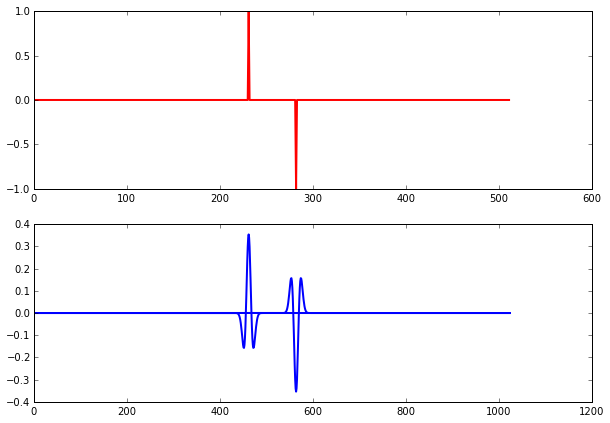Exercise 6

Plot the evolution of the criteria F, ERC and Coh as a function of $d$. Do the same plot for other signs patterns for $x_0$. Do the same plot for a Dirac comb with a varying spacing $d$.

In :
run -i nt_solutions/sparsity_6_l1_recovery/exo6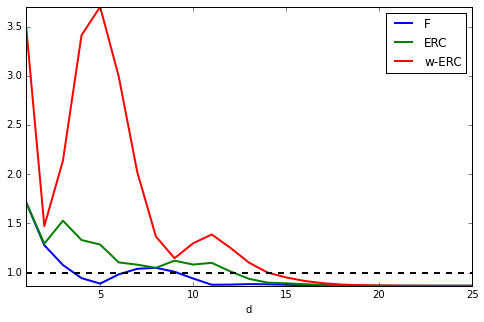In :
## Insert your code here.